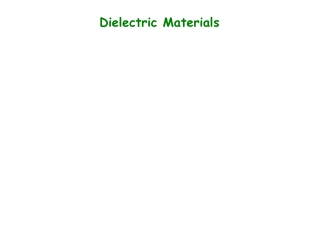DownloadDownload PresentationDielectric Materials

# Dielectric Materials

Download Presentation## Dielectric Materials

- - - - - - - - - - - - - - - - - - - - - - - - - - - E N D - - - - - - - - - - - - - - - - - - - - - - - - - - -
##### Presentation Transcript

1. Dielectric Materials

2. E What is a dielectric material? • Dielectric materials consist of polar molecules which • are normally randomly oriented in the solid. • They are not conductors. • When a dielectric material is placed in an external • electric field, the polar molecules rotate so they align with • the field. This creates an excess of positive charges on • one face of the dielectric and a corresponding excess • of negative charges on the other face.

3. Dielectric Material is smaller in many materials than it would be in a vacuum for the same arrangement of charges. Eg. Parallel plates: Dielectric material Eo Ei + + + + Net field: E=Eo-Ei This makes the potential difference smaller (V=Ed) between the parallel plates of the capacitor for the same charges on the plates and thus capacitance is larger, since Q=C/V.

4. Dielectric Constant (“kappa”) = “dielectric constant” = (a pure number ≥ 1) So, (for parallel plates) Or Where C0 is the capacitance without the dielectric. Hence, the capacitance of a filled capacitor is greaterthan an empty one by a factor

5. Materialk Vacuum 1 (definition) air 1.0006 paper 3.7 glass ~4 – 6 polystyrene 2.6 water 80 For any geometry: i.e. Replace “e0” with “ ke0 ” in the formulae.

6. Example: A parallel-plate capacitor has plates of 2cm by 3cm in dimension. The plates are separated by a 1mm thickness of paper. Paper with a dielectric constant of 3.7 is inserted between the plates. • What is the capacitance of this capacitor?

7. Example:The inner conductor of a coaxial cable has a radius of 0.800 mm, and the outer conductor’s inside radius is 3.00 mm. The space between the conductors is filled with polyethylene, which has a dielectric constant of 2.30 and a dielectric strength of 18.0 × 106 V/m. What is the maximum potential difference that this cable can withstand?

8. Quiz: What are the induced charges on the top/bottom of the dielectric as it is pushed between the plates? • What happens to the amount of charge on each plate • as the dielectric material is pushed in between? • The charges on the plates increase • The charges on the plates decrease • No change in charges

9. Quiz Two oppositely charged spheres are suspended a distance d apart in a beaker of distilled water. The force between them is • the same as if they were in air • larger • smaller

10. Why use dielectrics in capacitors? • the capacitance is increased by a factor k • the dielectric material gives mechanical strength (holds the conductors apart) • the plate separation can be smaller (which also increases capacitance) : C=Q/V=Q/(Ed) • the “dielectric breakdown”, or maximum electric field before conduction between the plates starts, can be higher than for air, allowing higher voltage ratings

11. Dielectric Breakdown There is a maximum voltage that can be applied toa capacitor without causing a discharge. his voltage depends on the dielectric strength (maximum electric field intensity) of the dielectric. Eg: for air, this is 3x106 V/m For many materials the strengths are in the 106 V/m range.

12. Example Air breaks down at 3x106 V/m. Assuming that storm clouds are 1km above the earth, approximate the maximum charge that they can store (assume the cloud to be a 1km by 1km rectangle).

13. Summary of Capacitance +Q on one conductor, -Q on the other conductor Potential difference between them • Deriving Formulae: (for plates, spheres, cylinders) • Assume charges +Q, -Q • Calculate E from Gauss’s Law • Integrate along a field line: